## ISC Maths Previous Year Question Paper 2018 Solved for Class 12

Time Allowed: 3 Hours
Maximum Marks: 100

(Candidates are allowed additional 15 minutes for only reading the paper. They must NOT start writing during this time.)

• The Question Paper consists of three sections A, B and C.
• Candidates are required to attempt all questions from Section A and all questions either from Section B or Section C.
• Section A: Internal choice has been provided in three questions of four marks each and two questions of six marks each.
• Section B: Internal choice has been provided in two questions of four marks each.
• Section C: Internal choice has been provided in two questions of four marks each.
• All working, including rough work, should be done on the same sheet as, and adjacent to the rest of the answer.
• The intended marks for questions or parts of questions are given in brackets [ ].
• Mathematical tables and graph papers are provided.

Section – A (80 Marks)

Question 1. [10 × 2]
(i) The binary operation * : R × R → R is defined as a * b = 2a + b. Find (2 *3) *4.
(ii) If A = $$\begin{pmatrix} 5 & a \\ b & 0 \end{pmatrix}$$ and A is symmetric matrix, show that a = b.
(iii) Solve : 3tan-1x + cot-1x = π
(iv) Without expanding at any stage, find the value of: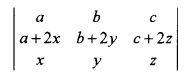(v) Find the value of constant ‘k’ so that the function f(x) defined as: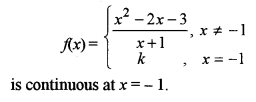(vi) Find the approximate change in the volume ‘V’ of a cube of side x metres caused by decreasing the side by 1%.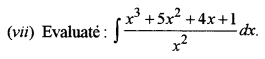(viii) Find the differential equation of the family of concentric circles x2 + y2 = a2.
(ix) If A and B are events such that P(A) = $$\frac { 1 }{ 2 }$$, P(B) = $$\frac { 1 }{ 3 }$$ and P(A∩B) =$$\frac { 1 }{ 4 }$$, then find: (a) P(A/B) (b) P(B/A)
(x) In a race, the probabilities of A and B winning the race are $$\frac { 1 }{ 3 }$$ and $$\frac { 1 }{ 6 }$$ respectively. Find the probability of neither of them winning the race.
Solution:
(i) Given binary operation * : R × R → R is defined as:
a * b = 2a + b
(2 * 3) * 4 = [2(2) +3) * 4 = 7 * 4 = 2(7) + 4 = 18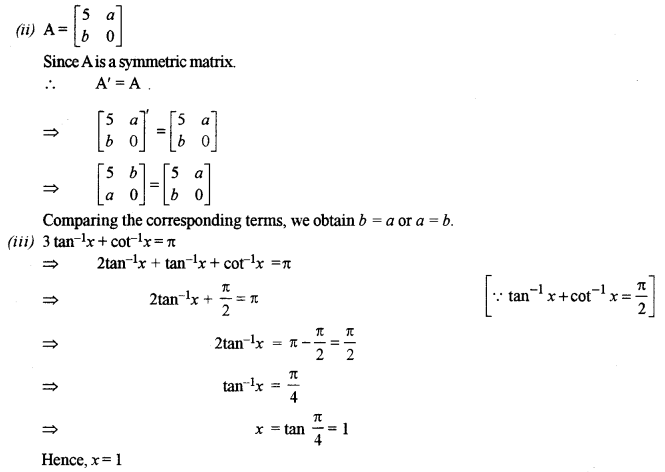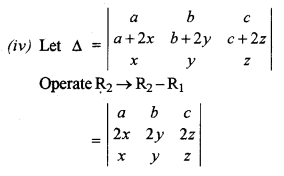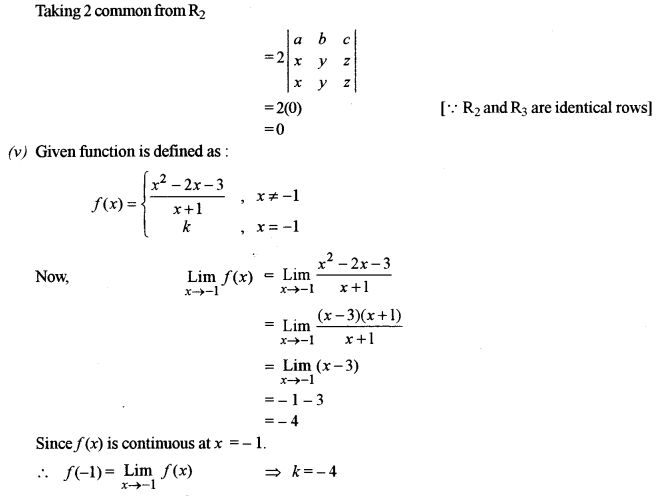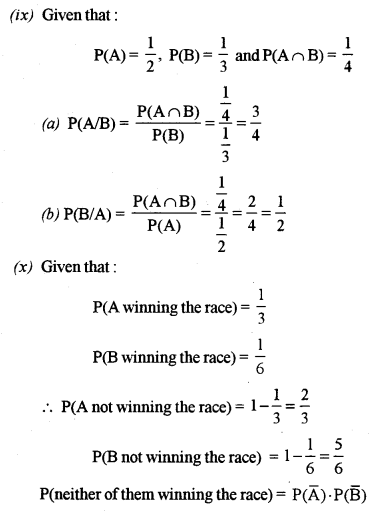Question 2. 
If the function f(x) = √(2x – 3) is invertible then find its inverse. Hence prove that (fof-1)(x) = x.
Solution: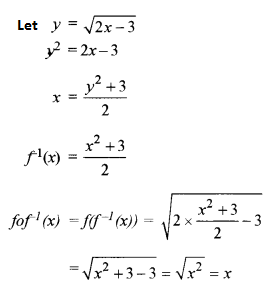Question 3. 
If tan-1a + tan-1b + tan-1c = π, prove that a + b + c = abc.
Solution: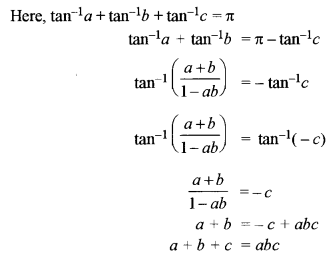Question 4. 
Use properties of determinants to solve for x: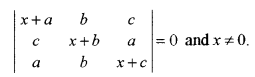Solution:Question 5.
(a) Show that the function $$f(x)=\left\{\begin{array}{ll}{x^{2}} & {, \quad x \leq 1} \\ {\frac{1}{x}} & {, \quad x>1}\end{array}\right.$$ is continuous at x = 1 but not differentiable.
Or
(b) Verify Rolle’s theorem for the following function:
f(x) = e-x sin x on [0, π]
Solution: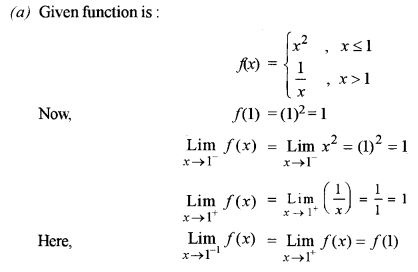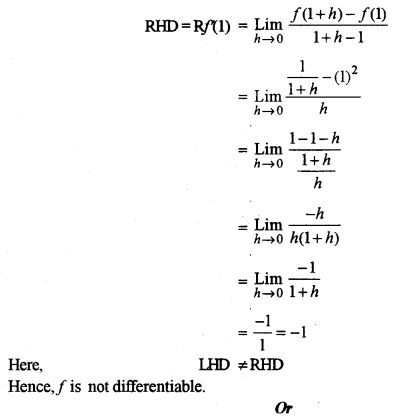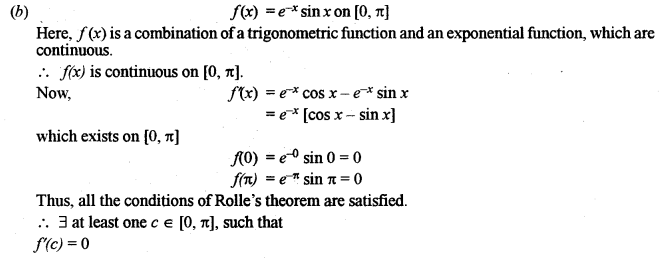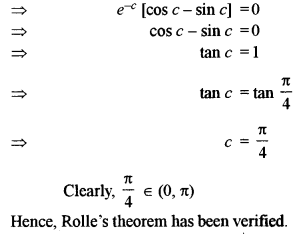Question 6.Solution: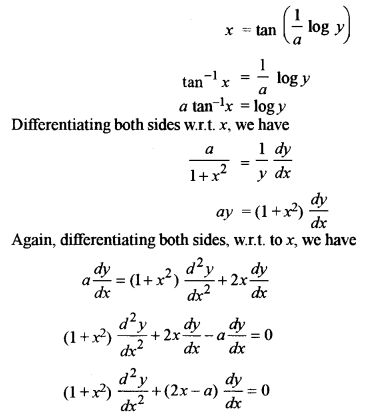Question 7.
Evaluate: ∫ tan-1√x dx 
Solution: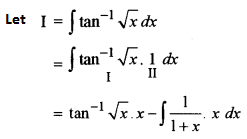Question 8. 
(a) Find the points on the curves = 4x3 – 3x + 5 at which the equation of the tangent is parallel to the x-axis.
Or
(b) Water is dripping out from a conical funnel of semi-vertical angle $$\frac { \pi }{ 4 }$$ at the uniform rate of 2 cm2/sec in the surface, through a tiny hole at the vertex of the bottom. When the slant height of the water level is 4 cm, find the rate of decrease of the slant height of the water.
Solution: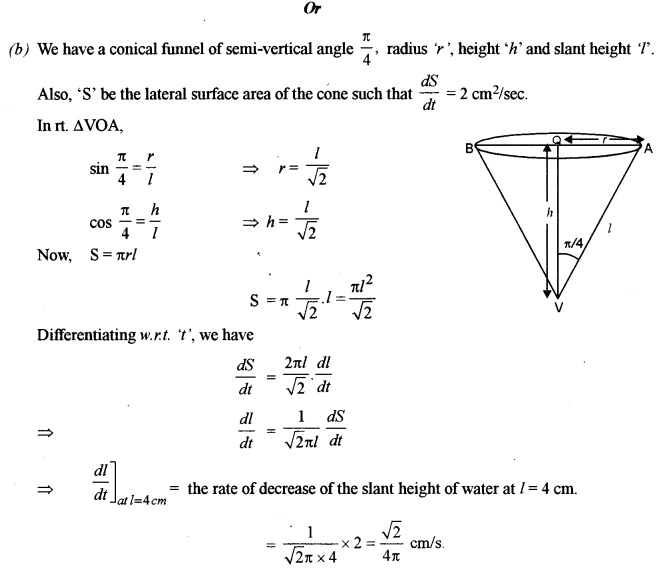Question 9.
(a) Solve: sin x $$\frac { dy }{ dx }$$ – y = sin x. tan$$\frac { x }{ 2 }$$.
or
(b) The population of a town grows at the rate of 10% per year. Using differential equation, find how long will it take for the population to grow 4 times.
Solution: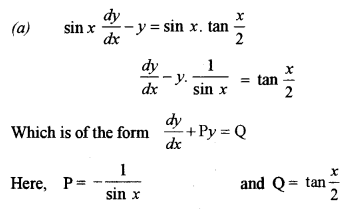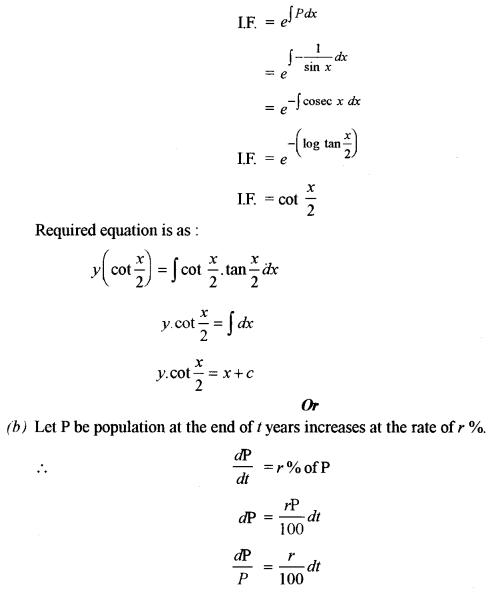Hence, the time required is 6.021 years.

Question 10.
(a) Using matrices, solve the following system of equations:
2x – 3y + 5z = 11
3x + 2y – 4z = -5
x + y – 2z = -3
Or
(b) Using elementary transformation, find the inverse of the matrix:
$$\left[ \begin{matrix} 0 & 1 & 3 \\ 1 & 2 & 3 \\ 3 & 1 & 1 \end{matrix} \right]$$
Solution:
(a) Given system of equations are:
2x – 3y + 5z = 11
3x + 2y – 4z = -5
x + y – 2z = -3
Corresponding matrix equation is:
AX = B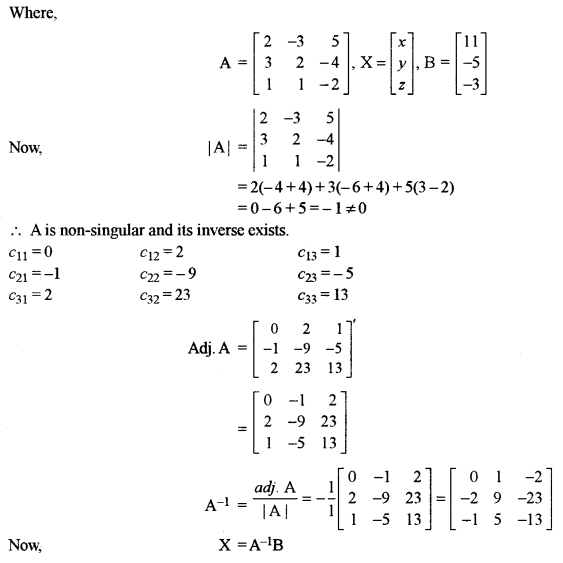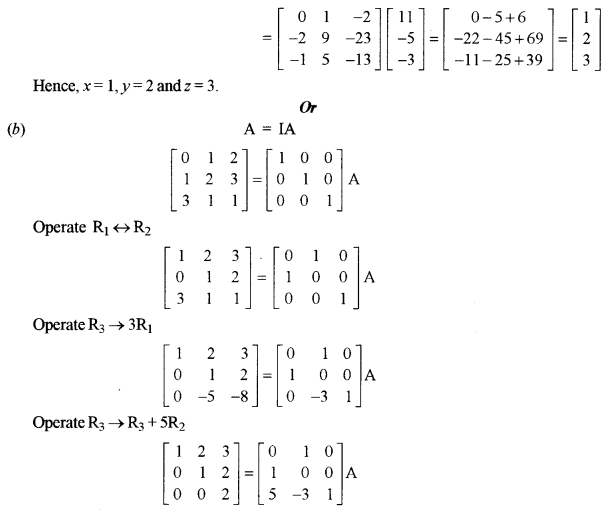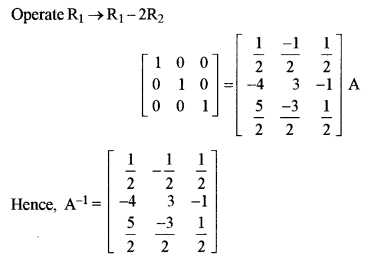Question 11. 
A speaks the truth in 60% of the cases, while B in 40% of the cases. In what per cent of cases are they likely to contradict each other in stating the same fact?
Solution:
Let E be the event of A speaking truth and F be the event of B speaking truth.
P(E) = $$\frac { 60 }{ 100 }$$ = $$\frac { 3 }{ 5 }$$, P(F) = $$\frac { 40 }{ 100 }$$ = $$\frac { 2 }{ 5 }$$
Probability of A and B likely to contradict each other in stating the same fact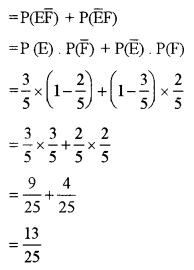Question 12.
A cone is inscribed in a sphere of radius 12 cm. If the volume of the cone is maximum, find its height.Solution:Question 13.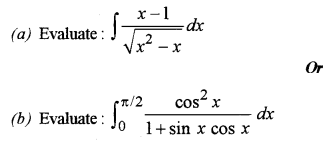Solution: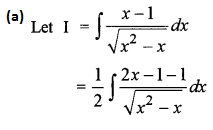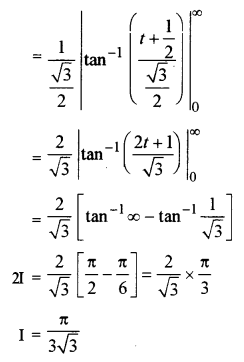Question 14. 
From a lot of 6 items containing 2 defective items, a sample of 4 items are drawn at random. Let the random variable X denote the number of defective items in the sample. If the sample is drawn without replacement, find:
(a) The probability distribution of X
(b) Mean of X
(c) Variance of X
Solution:
In a lot of 6 items, 2 items are defective. A sample of 4 items is drawn at random.
Let the random variable X denote the number of defective items in the sample.
X may have value 0, 1, 2Section – B (20 Marks)

Question 15. [3 × 2]
(a) Find λ if the scalar projection of $$\vec{a}=\lambda \hat{i}+\hat{j}+4 \hat{k} \text { on } \vec{b}=2 \hat{i}+6 \hat{j}+3 \hat{k}$$ is 4 units.
(b) The Cartesian equation of a line is: 2x – 3 = 3y + 1 = 5 – 6z. Find the vector equation of a line passing through (7, -5, 0) and parallel to the given line.
(c) Find the equation of the plane through the intersection of the planes $$\vec{r} \cdot(\hat{i}+3 \hat{j}-\hat{k})=9 \text { and } \vec{r} \cdot(2 \hat{i}-\hat{j}+\hat{k})=3$$ and passing through the origin.
Solution: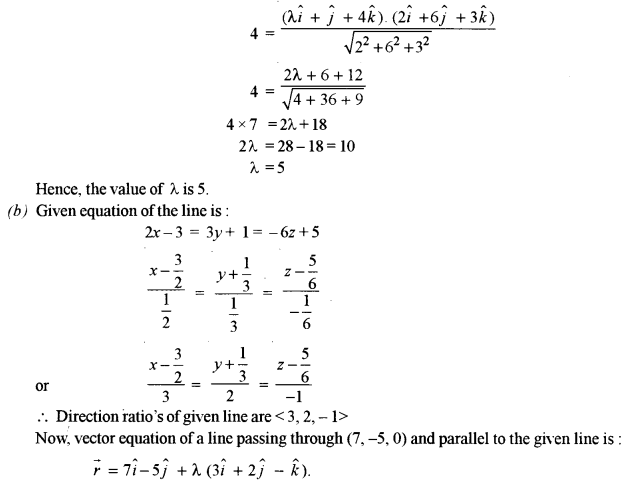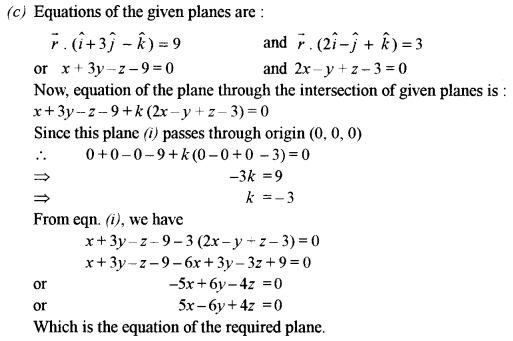Question 16. 
(a) If A, B, C are three non-collinear points with position vectors $$\vec{a}, \vec{b}, \vec{c}$$ respectively, then show that the length of the perpendicular from C on AB is $$\frac{|(\vec{a} \times \vec{b})+(\vec{b} \times \vec{c})+(\vec{c} \times \vec{a})|}{|\vec{b}-\vec{a}|}$$
Or
(b) Show that the four points A, B, C and D with position vectors $$4 \hat{i}+5 \hat{j}+\hat{k},-\hat{j}-\hat{k}, \hat{3} \hat{i}+9 \hat{j}+4 \hat{k} \text { and } 4(-\hat{i}+\hat{j}+\hat{k})$$ respectively, are coplanar.
Solution:
(a) Let h be the perpendicular distance from point C to the line segment AB.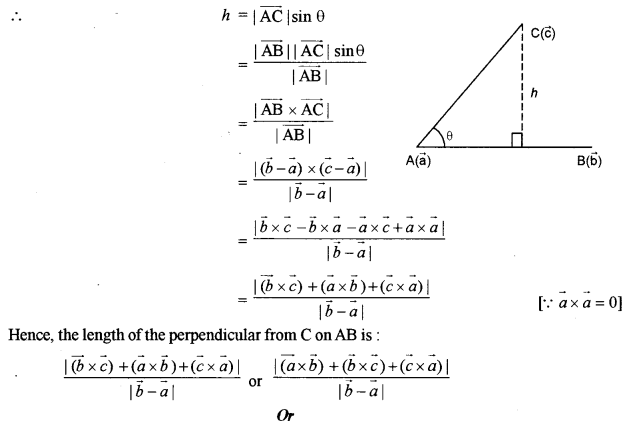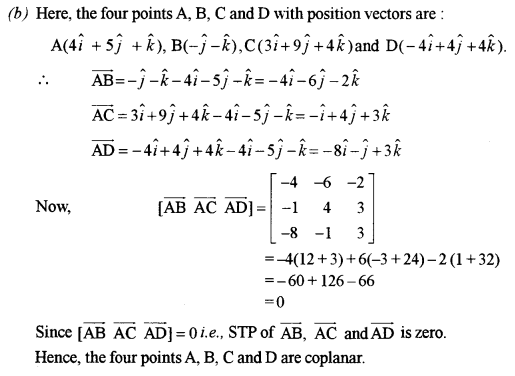Question 17. 
(a) Draw a rough sketch of the curve and find the area of the region bounded by curve y2 = 8x and the line x = 2.
Or
(b) Sketch the graph of y = |x + 4|. Using integration, find the area of the region bounded by the curve y = |x + 4| and x = -6 and x = 0.
Solution:
(a) Given curves are:
y2 = 8x …(i)
and x = 2 …(ii)
Putting x = 2 in eqn. (i),
we have y2 = 16
⇒ y = ±4
when x = 2, y = 4
when x = 2, y = -4
Points of intersections are (2, 4) and (2, -4)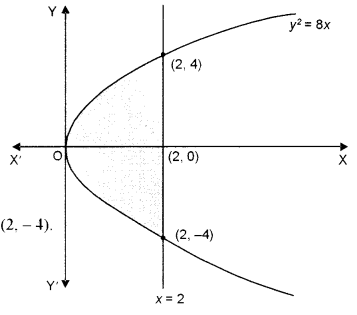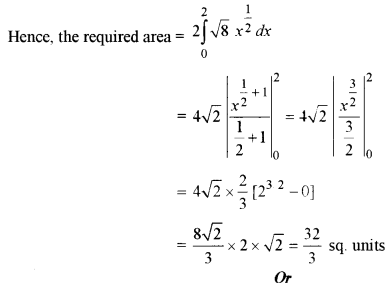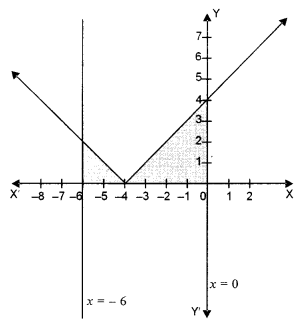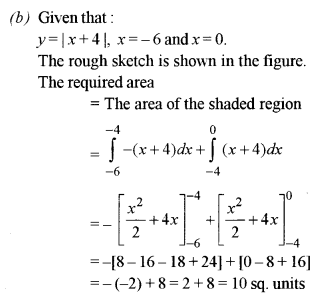Question 18.
Find the image of a point having position vector: $$3 \hat{i}-2 \hat{j}+\hat{k}$$ in the Plane $$\vec{r} \cdot(3 \hat{i}-\hat{j}+4 \hat{k})=2$$.
Solution:
Given point is P(3, -2, 1) and plane is 3x – y + 4z = 2.
D.R’s of normal to the plane are < 3, -1, 4 >
D.R’s of line PQ are <3, -1, 4 >
Equation of line PQ is, where Q is the foot of a perpendicular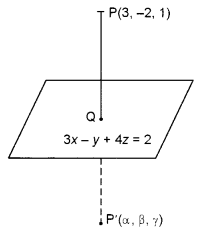Section – C (20 Marks)

Question 19. [3 × 2]
(a) Given the total cost function for x units of a commodity as:
C(x) = $$\frac { 1 }{ 3 }$$ x3 + 3x2 – 16x + 2.
Find:
(i) Marginal cost function
(ii) Average cost function
(b) Find the coefficient of correlation from the regression lines: x – 2y + 3 = 0 and 4x – 5y + 1 = 0.
(c) The average cost function associated with producing and marketing x units of an item is given by AC = 2x – 11 + $$\frac { 50 }{ x }$$. Find the range of values of the outputx, for which AC is increasing.
Solution:
(a) Given the total cost function for x units of a commodity is:
C(x) = $$\frac { 1 }{ 3 }$$ x3 + 3x2 – 16x + 2
(i) C'(x) = x2 + 6x – 16
Which is the required marginal cost function
(ii) Average cost function = $$\frac { C(x) }{ x }$$
$$\frac { 1 }{ 3 }$$ x + 3x – 16 + $$\frac { 2 }{ x }$$
(b) Given regression lines are:
x – 2y + 3 = 0 …..(i)
and 4x – 5y + 1 = 0 …..(ii)
From eqn. (i), we have
x = 2y – 3
Reg. of x on y = 2
From eqn. (ii), we have(c) The average cost function associated with producing and marketing x units of an item is given as:
AC = 2x – 11 + $$\frac { 50 }{ x }$$
Output’for which AC increases is:
$$\frac { d }{ dx }$$(AC) > 0
⇒ $$\frac { d }{ dx }$$ (2x – 11 + $$\frac { 50 }{ x }$$) > 0
⇒ $$2-\frac{50}{x^{2}}>0$$
⇒ x2 – 25 > 0
⇒ (x – 5)(x + 5) > 0
⇒ x > 5 [∵ x > 0]
Clearly, the average cost increases, if the output x > 5.

Question 20.
(a) Find the line of regression of y on x from the following table.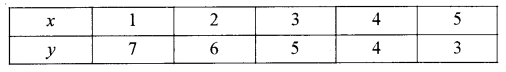Hence, estimate the value of y when x = 6.
Or
(b) From the given data: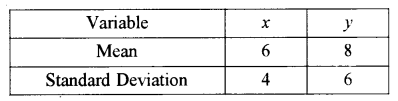and correlation coefficient: $$\frac { 2 }{ 3 }$$. Find:
(i) Regression coefficients byx and bxy
(ii) Regression line x on y
(iii) Most likely value of x when y = 14
Solution:
(a) Given that: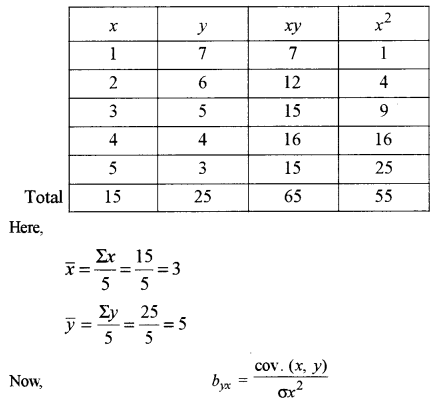Question 21.
(a) A product can be manufactured at a total cost $$C(x)=\frac{x^{2}}{100}+100 x+40$$, where x is the number of units produced. The price at which each unit can be sold is given by P = (200 – $$\frac { x }{ 400 }$$)
Determine the production level x at which the profit is maximum. What are the price per unit and total profit at the level of production?
Or
(b) A manufacturer’s marginal cost function is $$\frac{500}{\sqrt{2 x+25}}$$. Find the cost involved to increase production from 100 units to 300 units.
Solution: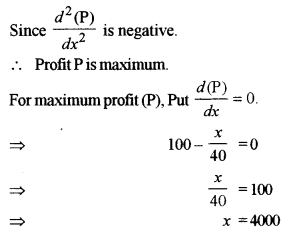Question 22.
A manufacturing company makes two types of teaching aids A and B of Mathematics for Class X. Each type of A requires 9 labour hours for fabricating and 1 labour hour for finishing. Each type of B requires 12 labour hours for fabricating and 3 labour hours for finishing. For fabricating and finishing, the maximum labour hours available per week are 180 and 30 respectively. The company makes a profit of ₹ 80 on each piece of type A and ₹ 120 on each piece of type B. How many pieces of type A and type B should be manufactured per week to get a maximum profit? Formulate this as Linear Programming Problem and solve it. Identify the feasible region from the rough sketch. 
Solution:
Let x and y be the number of teaching aids of type A and type B be produced by the company.
Objective Function (Z) = 80x + 120y
Subject to constraints
9x + 12y ≤ 180
or 3x + 4y ≤ 60,
x + 3y ≤ 30
and x, y ≥ 0
Table of solutions of 3x + 4y = 60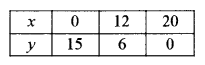Table of solutions of x + 3y = 30Plot the points A(0, 15), B(12, 6), C (20, 0), D(0, 10) and E(30, 0) to get the required graph as shown in the figure. Shaded region is the required feasible region and comer points of bounded feasible region are: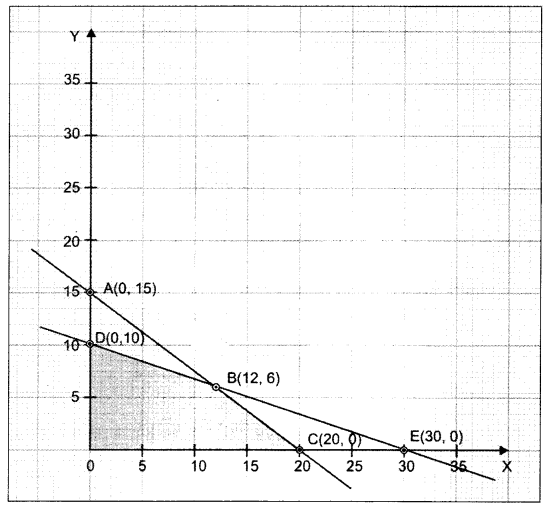O(0, 0), B(12, 6), C(20, 0) and D(0, 10)
At O(0, 0), Z = 0 + 0 = 0
At C(20, 0), Z = 20 × 80 + 0 = 1600
At B(12, 6), Z = 12 × 80 + 120 × 6 = 1680 → Maximum
At D(0, 10), Z = 0 + 120 × 10 = 1200
Hence, maximum profit can be made by manufacturing 12 teaching aids of type A and 6 teaching aids of type B.